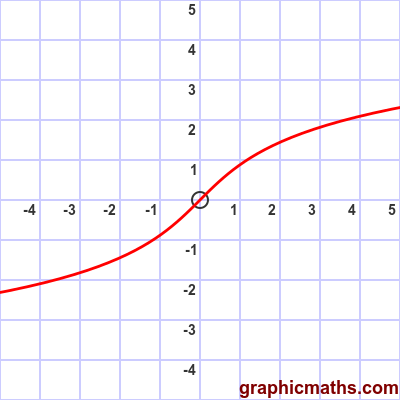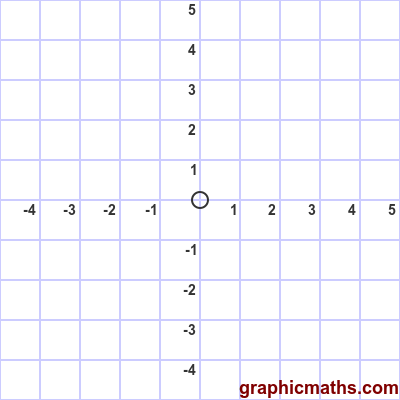# arsinh function

By Martin McBride, 2020-02-03
Tags: arsinh
Categories: hyperbolic functions

The arsinh function is a hyperbolic function. It is the inverse of the sinh, and is also known as the inverse hyperbolic sine function.

Why is it called the arsinh rather than the arcsinh? See here.

## Equation and graph

The arsinh function is defined as the inverse of sinh, ie if:

$$x = \sinh{y}$$

then:

$$y = \operatorname{arsinh}{x}$$

There is also a formula for finding arsinh as a logarithm, see below.

Here is a graph of the function:## arsinh as inverse of sinh

This animation illustrates the relationship between the sinh function and the arsinh function:The first, blue, curve is the sinh function.

The grey dashed line is the line $y=x$.

The second, red, curve is the arsinh function. As with any inverse function, it is identical to the original function reflected in the line $y=x$.

## Logarithm formula for arsinh

arsinh can be calculated directly, using a logarithm function, like this:

$$\operatorname{arsinh}{x}= \ln({x+{\sqrt {x^{2}+1}}})$$

Here is a proof of the logarithm formula for arsinh.

We will use:

$$u = \operatorname{arsinh}{x}$$

The sinh of u will be x, because sinh is the inverse of arsinh:

$$x = \sinh {u}$$

One form of the formula for sinh is:

$$\sinh{u} = \frac{e^{2u}-1}{2e^{u}}$$

This gives us:

$$x = \sinh {u} = \frac{e^{2u}-1}{2e^{u}}$$

Multiplying both sides by $2e^{u}$ gives:

$$2 x e^{u} = e^{2u} - 1$$

This is a quadratic in $e^{u}$ (using the fact that $e^{2u}=(e^{u})^2$):

$$0 = (e^{u})^2 -2 x (e^{u})-1$$

We use the quadratic formula with $a=1$, $b=-2x$, $c=-1$:

$$e^{u} = \frac{-b\pm\sqrt{b^2-4ac}}{2a} = \frac{2x\pm\sqrt{4{x^2}+4}}{2}$$

Simplifying and taking the positive solution (since $e^{u}$ must be positive) gives:

$$e^{u} = x+\sqrt{x^2+1}$$

Taking the logarithm of both sides gives:

$$ln(e^{u}) = u = ln(x+\sqrt{x^2+1})$$

And since u is $\operatorname{arsinh}{x}$ this gives:

$$\operatorname{arsinh}{x}= \ln({x+{\sqrt {x^{2}+1}}})$$